Courses

# NCERT Exemplar - Waves (Part - 2) Notes | EduRev

## JEE : NCERT Exemplar - Waves (Part - 2) Notes | EduRev

The document NCERT Exemplar - Waves (Part - 2) Notes | EduRev is a part of the JEE Course NEET Revision Notes.
All you need of JEE at this link: JEE

Q.1. A steel wire has a length of 12 m and a mass of 2.10 kg. What will be the speed of a transverse wave on this wire when a tension of 2.06 × 104N is applied?
Ans.
Given, length of the wire l = 12 m
Mass of wire m = 2.10 kg
Tension in wire T = 2.06 x 104 N
Speed of transverse wave v =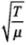where μ = Linear mass density  =  Mass per unit length,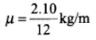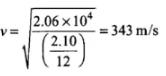Q.2. A pipe 20 cm long is closed at one end. Which harmonic mode of the pipe is resonantly excited by a source of 1237.5 Hz ?(sound velocity in air = 330 m s-1)
Ans.
Length of pipe, l = 20 cm = 20 x  10-2 m
Fundamental frequency of closed organ pipe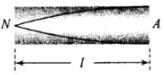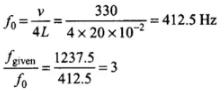It means third harmonic node of the pipe is resonantly excited by the source of given frequency.

Q.3. A train standing at the outer signal of a railway station blows a whistle of frequency 400 Hz still air. The train begins to move with a speed of 10 m s-1 towards the platform. What is the frequency of the sound for an observer standing on the platform? (sound velocity in air = 330 m s-1)
Ans.
v0 = 400 Hz
vz = 10 m/s
Velocity of sound in air va = 330 m/s
Apparent frequency by observer standing on platform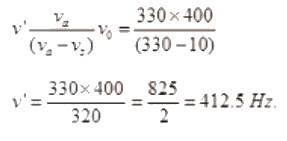Q.4. The wave pattern on a stretched string is shown in Figure Interpret what kind of wave this is and find its wavelength.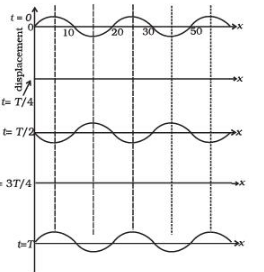Ans. If we observe the graph there are some points on the graph which are always at rest. The points on positions x = 10,20,30,40 never move, always at mean position with respect to time. These are forming nodes which characterize a stationary wave.
We know the distance between two successive nodes is equal to λ/2
λ = 2 x (node to node distance)
= 2 x (20 - 10) = 20 cm

Q.5. The pattern of standing waves formed on a stretched string at two instants of time are shown in Figure. The velocity of two waves superimposing to form stationary waves is 360 ms-1 and their frequencies are 256 Hz.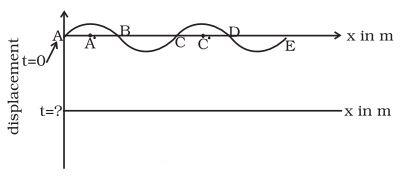(a) Calculate the time at which the second curve is plotted.
(b) Mark nodes and antinodes on the curve.
(c) Calculate the distance between A′ and C′ .
Ans.
Given frequency of the wave v = 256 Hz
∴ T = 1/v = 1/256 second = 0.00390
T = 3.9 x 10-3 seconds.
(a) In stationary wave a particle passes through it’s mean position after ever T/4 time
∴ In IInd curve displacement of all medium particle, are zero so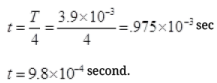(b) Point does not vibrate i.e., their displacement is zero always so nodes are at A, B, C, D and E. The point A’ and C’ are at maximum displacement so there are anti-nodes at A’ and C’.
Between A and C =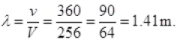Q.6. A tuning fork vibrating with a frequency of 512 Hz is kept close to the open end of a tube filled with water. The water level in the tube is gradually lowered. When the water level is 17 cm below the open end, maximum intensity of sound is heard. If the room temperature is 20° C, calculate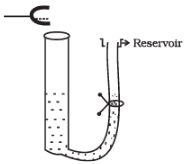(a) speed of sound in air at room temperature
(b) speed of sound in air at 0° C
(c) if the water in the tube is replaced with mercury, will there be any difference in your observations?
Ans.
If a pipe partially filled with water whose upper surface of the water acts as a reflecting surface of a closed organ pipe. If the length of the air column is varied until its natural frequency equals the frequency of the fork, then the column resonates and emits a loud note.
The frequency of tuning fork, f= 512 Hz.
For observation of first maxima of intensity,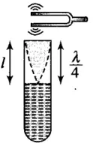The frequency of tuning fork, f  = 512 Hz.
For observation of first maxima of intensity,
(a) For first maxima of intensity, the length of the air column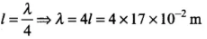Hence speed of sound v = fλ = 512 x (4 x 17 x 10-2)
= 348.16 m/s
(b) We know that v ∝ √T
where temperature (T) is in kelvin.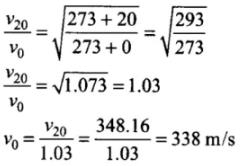(c) The resonance will still be observed for 17 cm length of air column above mercury. However, due to more complete reflection of sound waves at mercury surface, the intensity of reflected sound increases.

Q.7. Show that when a string fixed at its two ends vibrates in 1 loop, 2 loops, 3 loops and 4 loops, the frequencies are in the ratio 1 : 2 : 3 : 4.
Ans.
Let n be the number of loops in the string.
The length of each loop is λ/2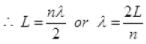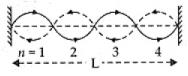v = vλ and λ = u/v.
So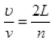v = n/2L. (v) v is stretch string =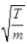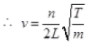For n = 1,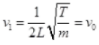If n = 2 then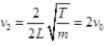n = 3 then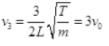∴ v1 : v2 : v3 : v4 = n1 : n2 : n3 : n4 = 1 : 2 : 3 : 4

Q.1. The earth has a radius of 6400 km. The inner core of 1000 km radius is solid. Outside it, there is a region from 1000 km to a radius of 3500 km which is in molten state. Then again from 3500 km to 6400 km the earth is solid. Only longitudinal (P) waves can travel inside a liquid. Assume that the P wave has a speed of 8 km s–1 in solid parts and of 5 km s–1 in liquid parts of the earth. An earthquake occurs at some place close to the surface of the earth. Calculate the time after which it will be recorded in a seismometer at a diametrically opposite point on the earth if wave travels along diameter?
Ans.
r= 1000 km
r2 = 3500 km
r3 = 6400 km
d1 = 1000km
d2 = 3500 - 1000 =2500 km
d3 = 6400 - 3500 = 2900 km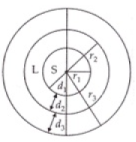Solid distance diametrically
= 2(d1 + d3) = 2(1000 +2900)
2 x 3900 km
Time taken by wave produced by earthquake in solid part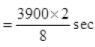Liquid part along diametrically = 2d2 = 2 x 2500
∴ Time taken by seismic  wave in  liquid part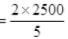Total time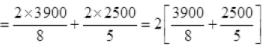= 2[487.5 + 500] = 2 x 987.5 = 1975 sec.
= 32 min 55 sec.

Q.2. If c is r.m.s. speed of molecules in a gas and v is the speed of sound waves in the gas, show that c/v is constant and independent of temperature for all diatomic gases.
Ans.
We know that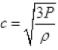for molecules.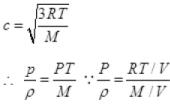M = molar mass of gas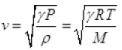∵ PV = nRT
n = 1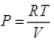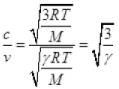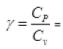adiabatic constant for diatomic gas
γ = 7/5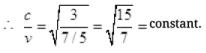Q.3. Given below are some functions of x and t to represent the displacement of an elastic wave.
(a) y = 5 cos (4x ) sin(20t)
(b) y = 4 sin(5x – t/2) + 3 cos (5x – t/2)
(c) y = 10 cos [(252 – 250) πt ] cos [(252+250)πt ]
(d) y = 100 cos (100πt + 0.5x )
State which of these represent
(a) a travelling wave along –x direction
(b) a stationary wave
(c) beats
(d) a travelling wave along +x direction
Ans.
(a) A travelling wave along (-x) direction must have + kx i.e., in
(iv) V = 100 cos (100πt + 0.5x) so (a) (iv).
(b) A stationary wave of the for y = 5 cos (4x) sin 20t is a stationary wave so (b) (i).
(c) Beats involve (v1 + v2) and (v1 - v2) so beats can be represented by
y = 10 cos [ (252 - 250)πt] represents beat so (c) (iii).
(d)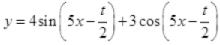Let 4 = acosϕ  ...(ii) and 3 = asinϕ   ...(iii)
acos2ϕ + a2sin2ϕ= 42 + 32 squaring and adding (ii), (iii)
a2 = 25 K
⇒ a = 5
Substituting (ii), (iii) in (i)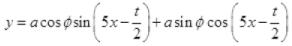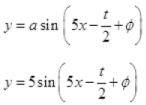Which represents the progressive wave in + x direction as the sign of kx (or 5x) and ωt(1/2 (t)) are opposite so it travels in + x direction. So (d) (ii)

Q.4. In the given progressive wave
y = 5 sin (100πt – 0.4πx )
where y and x are in m, t is in s. What is the
(a) amplitude
(b) wave length
(c) frequency
(d) wave velocity
(e) particle velocity amplitude
Ans.
Standard form of progressive wave travelling in +x direction (kx and ωt have opposite sign is given)
Eqn. is y = a sin (ωt - kx + ϕ)
y = 5 sin(100πt - 0.4πx + 0)
(a) Amplitude a = 5m
(b) Wavelength λ, k = 2π/λ
k = 0.4π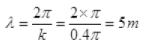(c) Frequency v, ω = 2 πv ⇒ v = ω/2π ∵ ω = 100π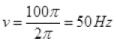(d) Wave velocity v = vλ = 50 x 5 = 250 m/s
(e) Particle (medium) velocity in the direction of amplitude at a distance from source.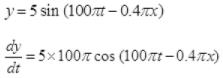For maximum velocity of particle is at its mean position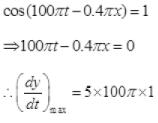vmax of medium particle = 500π m/s.

Q.5. For the harmonic travelling wave y = 2 cos 2π (10t–0.0080x + 3.5) where x and y are in cm and t is second. What is the phase difference between the oscillatory motion at two points separated by a distance of
(a) 4 m
(b) 0.5 m
(c) λ/2
(d) 3λ/4 (at a given instant of time)
(e) What is the phase difference between the oscillation of a particle located at x = 100cm, at t = T s and t = 5 s?
Ans.
y = 2 cos 2π (10t - 0.0080 x + 3.5)
y = 2 cos (20πt - 0.016πx + 7.0π)
Wave is propagated in +x direction because ωt and kx are in with opposite sign standard equation y = acos (ωt - kx + ϕ)
a = 2, ω = 20π, k = 0.016π and ϕ = 7π
(a) path difference p = 4 m (given) = 400 cm
Phase difference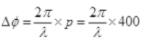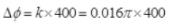Phase difference Δϕ = 6.4π rad.
(b) Path difference p = 0.5 m = 50 cm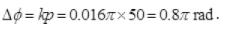(c) Path difference p = λ/2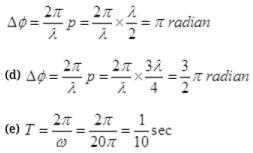x = 100 cm
t = T
At x = 100, t = T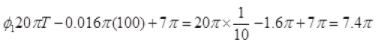At t = 5s
ϕ2 = 20π(5) = 0.016π(100) + 7π = 100π - 1.6π + 7π = 105.4π

ϕ- ϕ1=105.4π - 7.4π = 98π radian

Offer running on EduRev: Apply code STAYHOME200 to get INR 200 off on our premium plan EduRev Infinity!

,

,

,

,

,

,

,

,

,

,

,

,

,

,

,

,

,

,

,

,

,

;